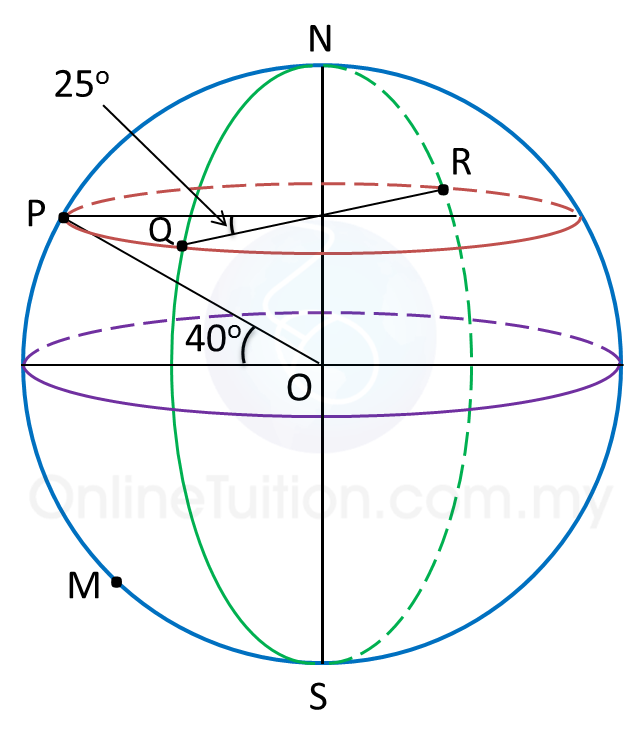# 9.6 SPM Practice (Long Questions)

Question 1:
Diagram below shows four points P, Q, R and M, on the surface of the earth. lies on longitude of 70oW. QR is the diameter of the parallel of latitude of 40o N. M lies 5700 nautical miles due south of P.(a) Find the position of R.

(b)
Calculate the shortest distance, in nautical miles, from Q to R, measured along the surface of the earth.

(c)
Find the latitude of M.

(d)
An aeroplane took off from R and flew due west to P along the parallel of latitude with an average speed of 660 knots.

Calculate the time, in hours, taken for the flight.

Solution:
(a)
Latitude of = latitude of Q = 40o N
Longitude of = (70o – 25o) W = 45o W
Longitude of = (180o – 45o) E = 135o E
Therefore, position of R = (40o N, 135oE).

(b)
Shortest distance from Q to R
= (180 – 40 – 40) x 60
= 100 × 60
= 6000 nautical miles

(c)
$\begin{array}{l}\angle POM=\frac{5700}{60}\\ \text{}={95}^{o}\\ \therefore \text{Latitude of}M=\left({95}^{o}-{40}^{o}\right)S\\ \text{}={55}^{o}S\end{array}$

(d)
$\begin{array}{l}\text{Time taken =}\frac{\text{distance from}R\text{to}P}{\text{average speed}}\\ \text{}=\frac{\left(180-25\right)×60×\mathrm{cos}{40}^{o}}{660}\\ \text{}=\frac{155×60×\mathrm{cos}{40}^{o}}{660}\\ \text{}=10.79\text{hours}\end{array}$